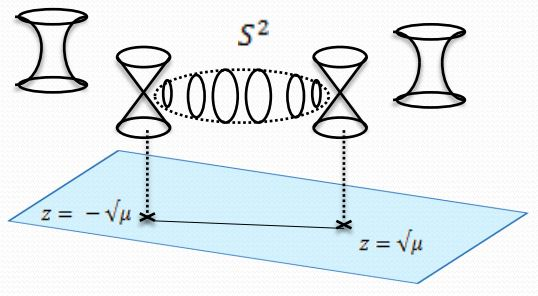# nLab (p,q)-string

Contents

under construction

## Surveys, textbooks and lecture notes

#### Gravity

gravity, supergravity

## Quantum theory

#### Quantum field theory

functorial quantum field theory

## Spin geometry

spin geometry

Dynkin labelsp. orth. groupspin grouppin groupsemi-spin group
SO(2)Spin(2)Pin(2)
B1SO(3)Spin(3)Pin(3)
D2SO(4)Spin(4)Pin(4)
B2SO(5)Spin(5)Pin(5)
D3SO(6)Spin(6)
B3SO(7)Spin(7)
D4SO(8)Spin(8)SO(8)
B4SO(9)Spin(9)
D5SO(10)Spin(10)
B5SO(11)Spin(11)
D6SO(12)Spin(12)
$\vdots$$\vdots$
D8SO(16)Spin(16)SemiSpin(16)
$\vdots$$\vdots$
D16SO(32)Spin(32)SemiSpin(32)

string geometry

# Contents

## Idea

In type II string theory: a bound state of $p$-F1-branes and $q$ D1-branes.

Under S-duality the modular group $SL_2(\mathbb{Z})$ acts on these $(p,q)$-tuples and mixes the $F1$- and the $D1$-brane states.

from M-branes to F-branes: superstrings, D-branes and NS5-branes

M-theory on $S^1_A \times S^1_B$-elliptic fibrationKK-compactification on $S^1_A$type IIA string theoryT-dual KK-compactification on $S^1_B$type IIB string theorygeometrize the axio-dilatonF-theory on elliptically fibered-K3 fibrationduality between F-theory and heterotic string theoryheterotic string theory on elliptic fibration
M2-brane wrapping $S_A^1$double dimensional reduction $\mapsto$type IIA superstring$\mapsto$type IIB superstring$\mapsto$$\mapsto$heterotic superstring
M2-brane wrapping $S_B^1$$\mapsto$D2-brane$\mapsto$D1-brane$\mapsto$
M2-brane wrapping $p$ times around $S_A^1$ and $q$ times around $S_B^1$$\mapsto$$p$ strings and $q$ D2-branes$\mapsto$(p,q)-string$\mapsto$
M5-brane wrapping $S_A^1$double dimensional reduction $\mapsto$D4-brane$\mapsto$D5-brane$\mapsto$
M5-brane wrapping $S_B^1$$\mapsto$NS5-brane$\mapsto$NS5-brane$\mapsto$$\mapsto$NS5-brane
M5-brane wrapping $p$ times around $S_A^1$ and $q$ times around $S_B^1$$\mapsto$$p$ D4-brane and $q$ NS5-branes$\mapsto$(p,q)5-brane$\mapsto$
M5-brane wrapping $S_A^1 \times S_B^1$$\mapsto$$\mapsto$D3-brane$\mapsto$
KK-monopole/A-type ADE singularity (degeneration locus of $S^1_A$-circle fibration, Sen limit of $S^1_A \times S^1_B$ elliptic fibration)$\mapsto$D6-brane$\mapsto$D7-branes$\mapsto$A-type nodal curve cycle degeneration locus of elliptic fibration(Sen 97, section 2)SU-gauge enhancement
KK-monopole orientifold/D-type ADE singularity$\mapsto$D6-brane with O6-planes$\mapsto$D7-branes with O7-planes$\mapsto$D-type nodal curve cycle degeneration locus of elliptic fibration(Sen 97, section 3)SO-gauge enhancement
exceptional ADE-singularity$\mapsto$$\mapsto$$\mapsto$exceptional ADE-singularity of elliptic fibration$\mapsto$E6-, E7-, E8-gauge enhancement

(e.g. Johnson 97, Blumenhagen 10)

brane intersections/bound states/wrapped branes/polarized branes

S-duality$\,$bound states:

intersecting$\,$M-branes:

• Igor Bandos, Superembedding Approach and S-Duality. A unified description of superstring and super-D1-brane, Nucl.Phys.B599:197-227,2001 (arXiv:hep-th/0008249)